Test: Linear Algebra- 1

# Test: Linear Algebra- 1

Test Description

## 20 Questions MCQ Test GATE Computer Science Engineering(CSE) 2023 Mock Test Series | Test: Linear Algebra- 1

Test: Linear Algebra- 1 for Computer Science Engineering (CSE) 2022 is part of GATE Computer Science Engineering(CSE) 2023 Mock Test Series preparation. The Test: Linear Algebra- 1 questions and answers have been prepared according to the Computer Science Engineering (CSE) exam syllabus.The Test: Linear Algebra- 1 MCQs are made for Computer Science Engineering (CSE) 2022 Exam. Find important definitions, questions, notes, meanings, examples, exercises, MCQs and online tests for Test: Linear Algebra- 1 below.
Solutions of Test: Linear Algebra- 1 questions in English are available as part of our GATE Computer Science Engineering(CSE) 2023 Mock Test Series for Computer Science Engineering (CSE) & Test: Linear Algebra- 1 solutions in Hindi for GATE Computer Science Engineering(CSE) 2023 Mock Test Series course. Download more important topics, notes, lectures and mock test series for Computer Science Engineering (CSE) Exam by signing up for free. Attempt Test: Linear Algebra- 1 | 20 questions in 60 minutes | Mock test for Computer Science Engineering (CSE) preparation | Free important questions MCQ to study GATE Computer Science Engineering(CSE) 2023 Mock Test Series for Computer Science Engineering (CSE) Exam | Download free PDF with solutions
 1 Crore+ students have signed up on EduRev. Have you?
Test: Linear Algebra- 1 - Question 1

### Let A be an mxn matrix and B an n*m matrix. It is given that determinant (Im + AB) = determinant (In + BA) , where Ik is the k*k identity matrix. Using the above property, the determinant of the matrix given below is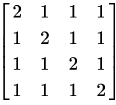Detailed Solution for Test: Linear Algebra- 1 - Question 1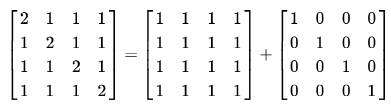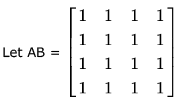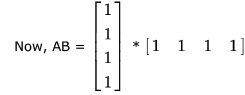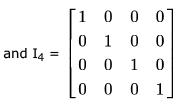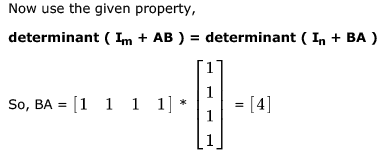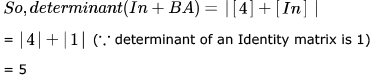Test: Linear Algebra- 1 - Question 2

### The determinant of the matrix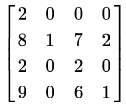Detailed Solution for Test: Linear Algebra- 1 - Question 2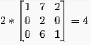Test: Linear Algebra- 1 - Question 3

### Which one of the following does NOT equal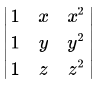?

Detailed Solution for Test: Linear Algebra- 1 - Question 3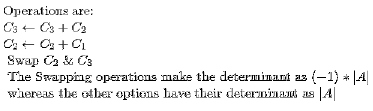Test: Linear Algebra- 1 - Question 4

The determinant of the matrix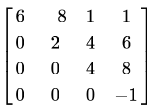Detailed Solution for Test: Linear Algebra- 1 - Question 4

As it's upper traingular matrix ... So determinant will be product of main diagonal element.
det(A) = 6*2*4* -1 = -48.
Similar concept can be appliead , if Matrix is lower triangular or Diagonal Matrix

Test: Linear Algebra- 1 - Question 5

The matrix of coefficients either have no solution or have infinite solutions is system of equations are

*Answer can only contain numeric values
Test: Linear Algebra- 1 - Question 6

If the matrix A is such that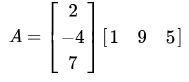then the determinant of  is equal to ______.

Detailed Solution for Test: Linear Algebra- 1 - Question 6

Hi,
For this kind of matrices Determinant is zero.
A will be a 3x3 matrix where the first row will be 2 [1 9 5], second row will be -4 [1 9 5] and third will be 7 [1 9 5]. That is, all the rows of A are linearly dependent which means A is singular.
When matrix is singular |A| = 0

*Answer can only contain numeric values
Test: Linear Algebra- 1 - Question 7

Two eigenvalues of a 3 x 3 real matrix P are (2 + √-1) and 3 . The determinant of P is _______

Detailed Solution for Test: Linear Algebra- 1 - Question 7

The determinant of a real matrix can never be imaginary. So, if one eigen value is complex, the other eigen value has to be its conjugate.

So, the eigen values of the matrix will be 2+i, 2-i and 3.

Also, determinant is the product of all eigen values.
So, the required answer is (2+i)*(2-i)*(3) = (4-i2)*(3) = (5)*(3) = 15.

*Answer can only contain numeric values
Test: Linear Algebra- 1 - Question 8

Suppose that the eigenvalues of matrix  A are 1,2, 4. The determinant of (A-1)T is _______________.

Detailed Solution for Test: Linear Algebra- 1 - Question 8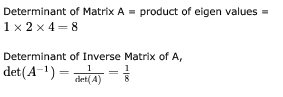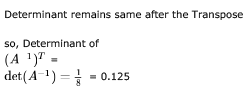Test: Linear Algebra- 1 - Question 9

In the given matrix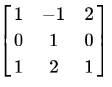, one of the eigenvalues is 1. The eigenvectors corresponding to the eigenvalue 1 are

Detailed Solution for Test: Linear Algebra- 1 - Question 9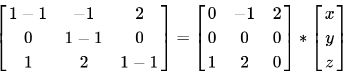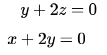now consider each of the triplets as the value of x, y, z and put in these equations the one which satisfies is the answer.
why so because an eigen vector represents a vector which passes through all the points which can solve these equations.
so we can observe that only option B is satisfying the equations.

Test: Linear Algebra- 1 - Question 10

Consider the following 2×2 matrix A where two elements are unknown and are marked by a and b. The eigenvalues of this matrix are -1 and 7. What are the values of a and b?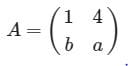Detailed Solution for Test: Linear Algebra- 1 - Question 10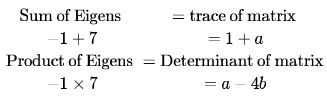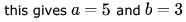*Answer can only contain numeric values
Test: Linear Algebra- 1 - Question 11

The value of the dot product of the eigenvectors corresponding to any pair of different eigenvalues of a 4 - by - 4 symmetric positive definite matrix is ___________

Detailed Solution for Test: Linear Algebra- 1 - Question 11

This is because eigen vectors corresponding to DIFFERENT eigen values of a REAL symmetric matrix are ORTHOGONAL to each other.
However, same eigen values they may not be.
And Dot -product of orthogonal vectors(perpendicular vectors ) is 0 (ZERO)

Test: Linear Algebra- 1 - Question 12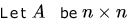real valued square symmetric matrix of rank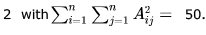Consider the following statements.

(I) One eigenvalue must be in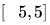(II) The eigenvalue with the largest magnitude must be strictly greater than 5

Which of the above statements about eigenvalues of  is/are necessarily CORRECT?

Detailed Solution for Test: Linear Algebra- 1 - Question 12

Eigen values of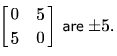Therefore second statement is false.
Since the rank of matrix  is 2, therefore atleast one eigen value would be zero for n>3.
For n= 2,  It can be proven that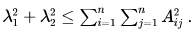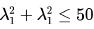Both  λ1 and λ2  would be real because  is a real symmetric matrix. Which implies that atleast one eigen value would be in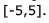Now, to prove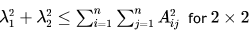matrix, let us consider the matrix is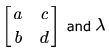is the eigen value
of this matrix.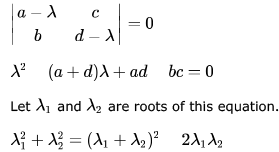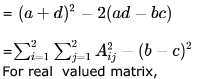(For real  symmetric matrix, b=c and < would be replaced by equal sign)

Test: Linear Algebra- 1 - Question 13

Let A be the matrix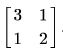What is the maximum value of xT Ax where the maximum is taken over all x that are the unit eigenvectors of A?

Detailed Solution for Test: Linear Algebra- 1 - Question 13

x = [x1 , x2] be a unit eigen vector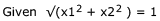i.e. x1 2 + x2 2 = 1
∴ { x is a unit Eigen vector}
x'Ax = x'Lx=  Lx'x  = L [x1,x2]' [x1,x2] = L [x12 + x22] = L(1) = L .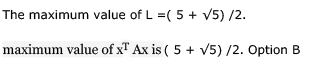Test: Linear Algebra- 1 - Question 14

Let A be a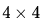matrix with eigen values -5,-2,1,4. Which of the following is an eigen value of  the matrix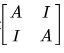, where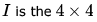identity matrix?

Detailed Solution for Test: Linear Algebra- 1 - Question 14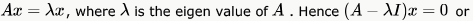So, for our given matrix, we have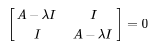This is a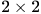block matrix where the first and last and the second and third elements are the same. So, applying the formulafor determinant of a block matrix as given here (second last case)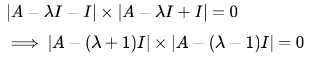Each of the eigen value of A is the solution of the equation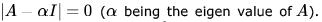. So, we can equate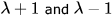to any of the eigen value of A, and that will get our value of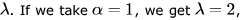and that is one of the choice. For no other choice, this equation holds. So, (c) 2 is the answer.

*Answer can only contain numeric values
Test: Linear Algebra- 1 - Question 15

Let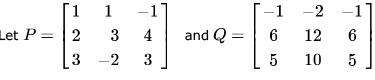be two matrices.

Then the rank of P + Q is ___________ .

Detailed Solution for Test: Linear Algebra- 1 - Question 15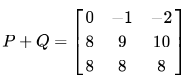det (P + Q), So Rank cannot be 3, but there exists a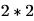submatrix such that determinant of submatrix is not 0.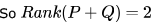Test: Linear Algebra- 1 - Question 16

Consider the matrix as given below.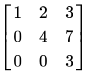Which one of the following options provides the CORRECT values of the eigenvalues of the matrix?

Detailed Solution for Test: Linear Algebra- 1 - Question 16

The given matrix is a upper triangular matrix and the eigenvalues of upper or lower traingular matrix are the diagonal values itself. (Property)

Test: Linear Algebra- 1 - Question 17

Which one of the following statements is TRUE about every  matrix with only real eigenvalues?

Detailed Solution for Test: Linear Algebra- 1 - Question 17

Trace is the sum of all diagonal elements of a square matrix.
Determinant of a matrix = Product of eigen values.
A) Is the right answer. To have the determinant negative ,atleast one eigen value has to be negative(but reverse may not be true). {you can take simple example with upper or lower triangular matrices. In the case option (b) , (c) and (d) reverse is always true .}

*Answer can only contain numeric values
Test: Linear Algebra- 1 - Question 18

The product of the non-zero eigenvalues of the matrix is ____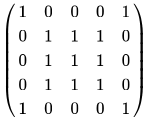Detailed Solution for Test: Linear Algebra- 1 - Question 18

We can see that the rank of the given matrix is 2 (since 3 rows are same, and other 2 rows are also same). Sum of eigen values = sum of diagonals. So, we have two eigen values which sum to 5. This information can be used to get answer in between the following solution. Let Eigen value be X. Now, equating the determinant of the following to 0 gives us the values for X. To find X in the following matrix, we can equate the determinant to 0. For finding the determinant we can use row and column additions and make the matrix a triangular one. Then determinant will just be the product of the diagonals which should equate to 0.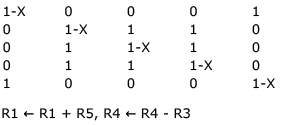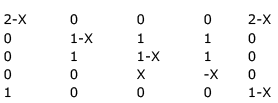Taking X out from R4, 2-X from R1, (so, X = 2 is one eigen value)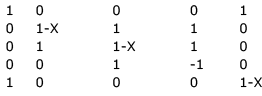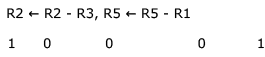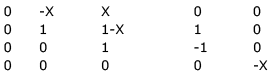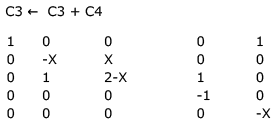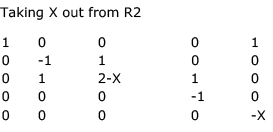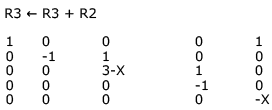Now, we got a triangular matrix and determinant of a triangular matrix is product of the diagonal.
So (3-X) (-X) = 0 => X = 3 or X = 0. So, X = 3 is another eigen value and product of non-zero eigen values = 2 * 3 = 6.

*Answer can only contain numeric values
Test: Linear Algebra- 1 - Question 19

If the characteristic polynomial of a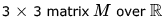(the set of real numbers) is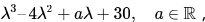and one eigenvalue of M is 2, then the largest among the absolute values of the eigenvalues of M is _______

Detailed Solution for Test: Linear Algebra- 1 - Question 19

Given that λ = 2 is an eigen value. So, it must satisfy characterstic equation.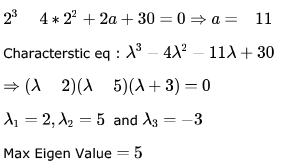*Answer can only contain numeric values
Test: Linear Algebra- 1 - Question 20

The larger of the two eigenvalues of the matrix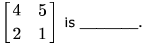Detailed Solution for Test: Linear Algebra- 1 - Question 20

For finding the Eigen Values of a Matrix we need to build the Characteristic equation which is of the form,

A - λI
Where A is the given Matrix.
λ is a constant

I is the identity matrix.
We'll have a Linear equation after solving A - λI. Which will give us 2 roots for λ.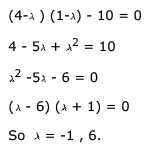6 is larger and hence is the Answer.

## GATE Computer Science Engineering(CSE) 2023 Mock Test Series

136 docs|165 tests
 Use Code STAYHOME200 and get INR 200 additional OFF Use Coupon Code
Information about Test: Linear Algebra- 1 Page
In this test you can find the Exam questions for Test: Linear Algebra- 1 solved & explained in the simplest way possible. Besides giving Questions and answers for Test: Linear Algebra- 1, EduRev gives you an ample number of Online tests for practice

## GATE Computer Science Engineering(CSE) 2023 Mock Test Series

136 docs|165 tests